# SQLite array | How array work in SQLite with Examples?

0
1## Definition on SQLite array

SQLite arrays are not directly supported as a data type for columns in SQLite databases. In relational databases generally, an array is used to store rows with different key columns as an array dimension that means more than one key for a particular key. But in SQLite we cannot directly implement arrays in SQLite. So we can use some other ways to encode the array concept in SQLie, we can use text file as an array that means we can import text file into the SQLite database or we can use foreign key constraint to implement the array in SQLite database.

Syntax:

create table specified table name (colm name 1 data type, colm name 2 data type, colm name 3 data type,……….colm name N data type, primary key (colm name 1, colm name 2, colm name 3));

Explanation

In the above syntax, we use create table statement to implement an array in SQLite database, here specified table name means actual table that we need to create, colm name 1, colm name 2 to colm name N is a column name that we need to create inside the table with the different data type. Here we also use the primary key constraint inside that we define the different keys and that are used as arrays in the SQLite database.

### How array work in SQLite?

Now let’s see how the array works in SQLite as follows.

Normally array is one type of data structure that is used to store the group of elements as per the user requirement, that means we need to implement an array in the database at that time array data structure uses the same data type to all stored elements that either integer or string. So let’s see the different ways to implement arrays in SQLite databases as follows.

In the first method we can use a text file as an array and import into the SQLite database. The text file means we can use the JSON array. JSON means JavaScript Object Notation and it is used to send data over the web page.
In another way, we can use foreign key concepts to implement the array in SQLite database. In which we can create multiple tables and that table stores the same kind of data like array data structure and we can access them by using the JOIN constraint as per the user requirement because the array we can directly implement in SQLite database.

### Examples

Now let’s see the different examples of JOIN to implement arrays in SQLite as follows.

First, create an emp with different attributes by using the following statement as follows.

`create table stud (stud_id integer primary key, stud_dept_id integer, stud_name text not null, stud_address text not null);`

Explanation

In above example, we use create table statement to create new table name as stud with different attributes such as stud_id with integer data type and primary key constraint, stud_dept_id with integer data type and primary key constraint, stud_name with text data type with not null constraint and stud_address with text data type with not null constraint. See here we define the primary key that is stud_id as shown in the above statement. End result of above statement as shown in below screenshot as follows.

`.table`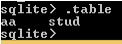Now insert some records into the stud by using the following statement as follows.

```Insert into stud (stud_id, stud_dept_id, stud_name, stud_address) values (1, 20, “JAY”, “Pune”), (2, 30, “Johan”, “Mumbai”), (3, 20, “Jenny”, “Mumbai”), (4, 20, “Sameer”, “Mumabi”), (5, 30, “Pooja”, “Pune”); select * from stud;```

Explanation

In the above statement, we use insert into statement to insert records into the stud table. End result of above statement as shown in below screenshot as follows.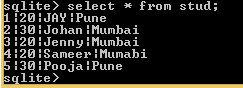Now create another table by using the create table statement as follows.

`create table info (info_id integer primary key, stud_last_name text not null, stud_id integer not null);`

Explanation

In above example, we use create table statement to create new table name as info with different attributes such as info_id with integer data type, stud_last_name with text data type and not null constraint and stud_id with integer data type and not null constraint as shown in above statement. End result of above statement as shown in below screenshot as follows.

`.table`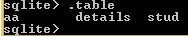Now insert new records into the details table by using the following statement as follows.

```Insert into info (info_id, stud_last_name, stud_id) values (11, “Sharma”, 1), (22, “Sinha”, 2), (33, “Mac”, 3), (44, “Gupta”, 4); select * from info;```

Explanation

By using the above statement we successfully insert new records. End result of above statement as shown in below screenshot as follows.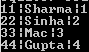Now use JOINS to implement the array as follows.

`select stud_name, stud_last_name from stud inner join info on stud.stud_id = info.stud_id;`

Explanation

In the above example, we use a select statement with inner join. See here we need to print student print student full name that is student first name and last name at that time we can use inner join to implement array in SQLite. End result of above statement as shown in below screenshot as follows.So we see how we can implement array data structure in SQLite by using alternate paths because in SQLite we cannot implement arrays directly.

Now see how to directly implement arrays in SQLite this is not the right way but till it shows results.

`create table aa (stud_id integer, email text [] [], test integer []);`

Explanation

In the above example we use create table statement to new table name as aa with different attributes, see here we define email and test as integer array data structure as shown in above statement.

After that insert some records into the demo table by using the following statement as follows.

```insert into aa values(‘{{“work”, “rah@gamil.com”}, {“other”, “aaa@gmail.com”}}’, ‘{85, 54, 45}’); select * from aa;```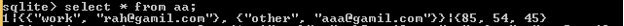Explanation

After insert operation, we see inserted records by using the select statement as follows. End result of above statement as shown in below screenshot as follows.

### Conclusion

We hope from this article you have understood about the SQLite array. From the above article, we have learned the basic syntax of array and we also see different examples of array. We also learned the rules of arrays. From this article, we learned how and when we use the SQLite array.

### Recommended Articles

This is a guide to SQLite array. Here we discuss the Introduction, syntax, How array work in SQLite, and examples. You may also have a look at the following articles to learn more –

The post SQLite array appeared first on EDUCBA.